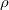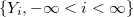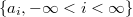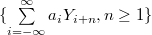ď»ż

### Limiting behaviour of moving average processes under$\rho$-mixing assumption

#### Abstract

Let$\{Y_i, -\infty be a doubly infinite sequence of identically distributed$\rho$-mixing random variables,$\{a_i,-\infty an absolutely summable sequence of real numbers. In this paper, we prove the complete convergence and Marcinkiewicz-Zygmund strong law of large numbers for the partial sums of the moving average processes$\{\sum\limits^\infty_{i=-\infty}a_i Y_{i+n},n\geq1\}$.

DOI Code: 10.1285/i15900932v30n1p17

Keywords:
moving average; Ď?-mixing; complete convergence; Marcinkiewicz-Zygmund strong laws of large numbers

Classification: 60F15

Full Text: PDF